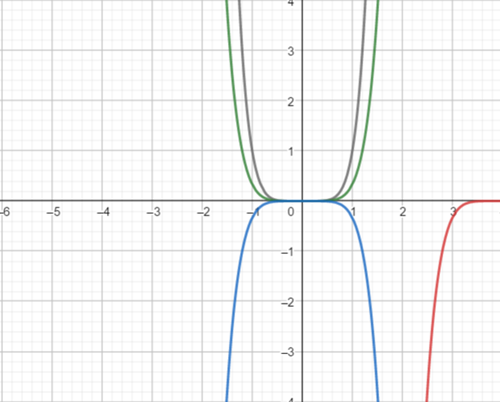# To graph the functionson the same screen and how they related to the graph in part (a). The functions are given below: ( a ) y 1 = x 6 ( b ) y 2 = 1 3 x 6 ( c ) y 3 = − 1 3 x 6 ( d ) y 4 = − 1 3 ( x − 4 ) 6### Precalculus: Mathematics for Calcu...

6th Edition
Stewart + 5 others
Publisher: Cengage Learning
ISBN: 9780840068071### Precalculus: Mathematics for Calcu...

6th Edition
Stewart + 5 others
Publisher: Cengage Learning
ISBN: 9780840068071

#### Solutions

Chapter 2.5, Problem 71E
To determine

## To graph the functionson the same screen and how they related to the graph in part (a).The functions are given below:  (a) y1=x6(b)y2=13x6(c)y3=−13x6(d)y4=−13(x−4)6

Expert Solution

### Explanation of Solution

Given information:

The function are:

(a) y1=x6(b)y2=13x6(c)y3=13x6(d)y4=13(x4)6

Graph :

the graph obtained as:Interpretation: from the above graph it is observed that for part(b) shrink the graph of part (a) vertically by a factor of 13 .

For part (c) shrink the graph of part (a) vertically by a factor of 13 and reflect in the x-axis .

For part (d) shift the graph of part (a) to the right 4 unit and shrink vertically by a factor of 13 then reflect in the x -axis.

### Have a homework question?

Subscribe to bartleby learn! Ask subject matter experts 30 homework questions each month. Plus, you’ll have access to millions of step-by-step textbook answers!